Algebra

Equations

Inequalities

Graphs

Numbers

Calculus

Matrices

Tutorials

# Division of Polynomials

Division of Polynomials

The following are some of the properties pertaining to fractions. These properties are discussed in Chapter 2.

1.  ab = acbc      2.   a+bc = ac+bc

3.  ab·cd = acbd    4.  ab÷cd = ab·dc

Note   Since division by zero is not deﬁned, all denominators are assumed different from zero

First we will discuss division of monomials, then division of a polynomial by a monomial, and ﬁnally division of two polynomials.

Division of Monomials

From the properties of fractions and the rules governing exponents we have

a8a5 = a5·a3a5·1 = a31 = a3

=a8-5

a4a4= 1

a7a10 = a7·1a7·a3 = 1a3

=1a10-7

THEOREM 4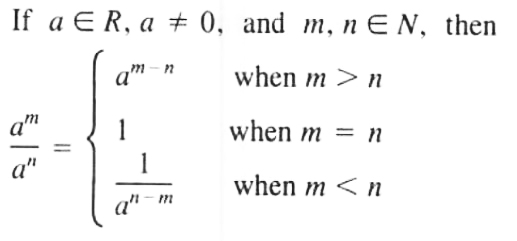Proof  aman = an·am-nan·1 = am-n  When m>n

aman = anan = 1        When m=n

aman = am·1am·an-m = 1an-m  When m <strong>EXAMPLE  </strong>1.  [[2^6/2^2 = 26-2 = 24

2.  a7a5 = a7-5 = a2

3.  (a-1)4(a-1)3 = (a-1)4-3 = (a-1)

4.  5454 = 1

5.  (x+1)3(x+1)3 = 1

6.  38312 = 1312-8 = 134

7.  a3a9 = 1a9-3 = 1a6

8.  (x+2)4(x+2)6 = 1(x+2)6-4 = 1(x+2)2

From the properties of fractions and the deﬁnition of exponents we have

(23)4 = 23·23·23·23

= 2·2·2·23·3·3·3

= 2434

Let's see how our Polynomial solver simplifies this and similar problems. Click on "Solve Similar" button to see more examples.

THEOREM 5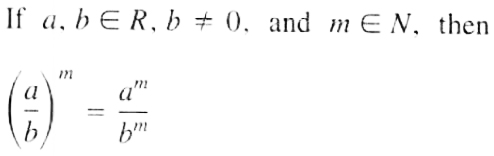Proof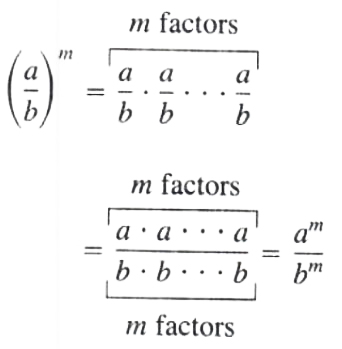COROLLARY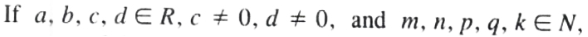then by the use of Theorem 2 and Theorem 3 and theorem 5, we have

(am bncp dq)k = (am bn)k(cp dq)k = amk bnkcpk dqk

EXAMPLE  Simplify 30 a3 b212 a2 b4 by applying the laws of exponents.

Solution  30 a3 b212 a2 b4 = (2·3·5 a3 b22·2·3 a2 b4)

= (2·32·2)·(52)·(a3a2)·(b2b4)

= (52)·(a1)·(1b2)

= (5 a2 b2)

Let's see how our Polynomial solver simplifies this and similar problems. Click on "Solve Similar" button to see more examples.

EXAMPLE  By applying the laws of exponents, simplify [2 x4 yz6 xy2]3

Solution We can simplify the fraction first before applying the outside exponent.

[2 x4 yz6 xy2]3 = [x3 z3 y]3 = x9 z333 y3 = x9 z327 y3

EXAMPLE  124183 = (22·3)4(2·32)3 = 28·3423·36

=(2823)·(3436) = (251)·(132) = 329

EXAMPLE  Simplify (2 a2 bc3)3(3 ab2)2 by applying the laws of exponents

Solution Here we cannot simplify ﬁrst. since the numerator and the denominator have different powers. Apply the outside exponents ﬁrst. then simplify,

(2 a2 bc3)3(3 ab2)2 = 23 a6 b3 c932 a2 b4 = 8 a4 c99 b

Let's see how our Polynomial solver simplifies this and similar problems. Click on "Solve Similar" button to see more examples.

EXAMPLE  Perform the indicated operations and simplify:

16 a4 b3÷( 2 ab)3 + 36 a5 b2÷( 3 a2 b)2

Solution 16 a4 b3÷( 2 ab)3 + 36 a5 b2÷( 3 a2 b)2

=16 a4 b3( 2 ab)3 + 36 a5 b2( 3 a2 b)2

=16 a4 b3 23 a3 b3 + 36 a5 b232 a4 b2

=16 a4 b3 8 a3 b3 + 36 a5 b29 a4 b2

= 2 a+4 a = 2 a

Division of a Polynomial by a Monomial

From the properties of fractions we have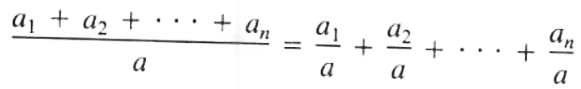Keep in mind that

a+bc means (a+b)÷cbut

a+ba = aa+ba = 1+ba

To divide a polynomial by a monomial, divide every term of the polynomial by the monomial.

EXAMPLE  Divide 12 x3-6 x2+18 x6 x and simplify.

Solution  12 x3-6 x2+18 x6 x = 12 x36 x+ 6 x26 x+18 x6 x

=2 x2-x+3

Let's see how our Polynomial solver simplifies this and similar problems. Click on "Solve Similar" button to see more examples.

EXAMPLE  Divide 3 a3-2 a2 b-ab2 ab and simplify.

Solution 3 a3-2 a2 b-ab2 ab = 3 a3 ab+ 2 a2 b ab+ ab2 ab

= (3 a2b)+2 a+b

EXAMPLE  Perform the indicated operations and simplify:

12 a4+4 a3-32 a24 a2 - (3 a-8) (a+1)

Solution 12 a4+4 a3-32 a24 a2 - (3 a-8) (a+1)

=(3 a2+a-8)-(3 a2-5 a-8)

=3 a2+a-8-3 a2+5 a+8 = 6 a

Division of Two Polynomials

Division is deﬁned as the inverse operation of multiplication; thus we start with a multiplication problem and then deduce the division operation.

(x2+3 x-5) (2 x-7) = x2 (2 x-7)+3 x (2 x-7)+( 5) (2 x-7)

=(2 x3-7 x2)+(6 x2-21 x)+( 10 x+35)

=2 x3-x2-31 x+35

Hence if (2 x3-x2-31 x+35) is divided by (2 x-7), the result is (x2+3 x-5), the first polynomial of multiplication problem.

The polynomial (2 x3-x2-31 x+35) is called the dividend, (2 x-7) is called the divisor, and (x2+3 x-5) is called the quotient. The first term of the dividend, 2 x3, comes from multiplying the first term of quotient, x2, by the first term of the divisor, 2 x. Thus to obtain the first term of quotient, x2, we divide the first term of the dividend , 2 x3 , by the first term of the divisor, 2 x. Multiplying the entire divisor (2 x-7) by that ﬁrst term of the quotient, x2, we get 2 x3-7 x2. Subtracting 2 x3-7 x2 from the dividend,

(2 x3-x2-31 x+35)-(2 x3-7 x2) = 6 x2-31 x+35

The quantity 6 x2-31 x+35 is our new dividend. The ﬁrst term, 6 x2, of the new dividend comes from multiplying the second term of the quotient, 3 x, by the first term of the divisor, 2 x. Thus to get the second term of the quotient, 3 x, divide the ﬁrst term of the new dividend, 6 x2, by the ﬁrst term of the divisor, 2 x. Multiplying the divisor (2 x-7) by the second term of the quotient, 3 x, we get 6 x2-21 x. Subtracting 6 x2-21 x from the new dividend,

(6 x2-31 x+35)-(6 x2-21 x) = 10 x+35

The quantity 10 x+35 is the new dividend. Dividing the first term of the new dividend ( 10 x) by the first term of the divisor, 2 x, we get the third term of the quotient ( 5). Multiplying the divisor (2 x-7) by the third term of the quotient ( 5), we get 10 x+35. Subtracting ( 10 x+35) from the dividend ( 10 x+35), We get zero.

Let us start the problem again an arrange it in a manner analogous to that of long division in arithmetic.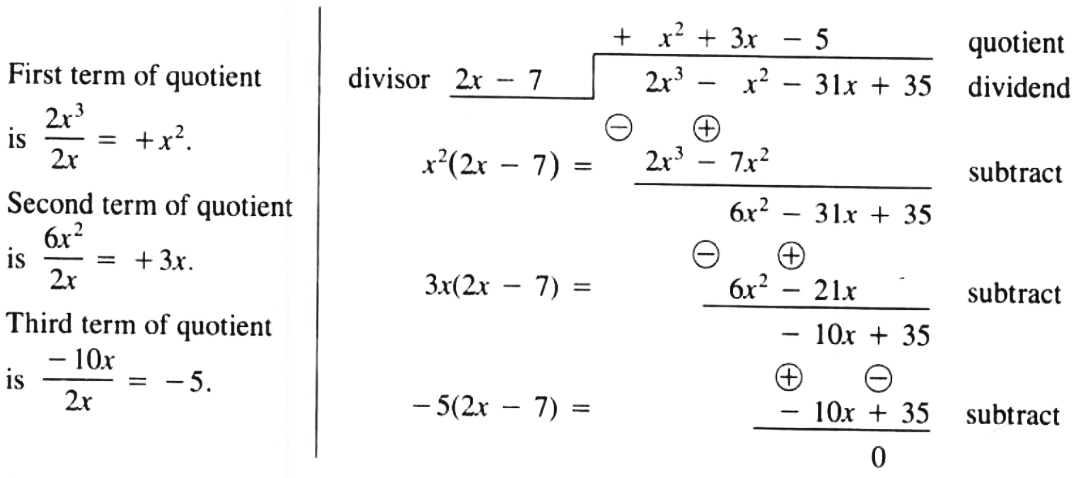Hence  2 x3-x2-31 x+352 x-7 = x2+3 x-5

DEFINITION  The degree of a polynomial in a literal number is the greatest exponent of that literal number in the polynomial.

EXAMPLE  x5 y-7 x4 y2-2 x3 y3+9 y4 is a polynomial of degree 5 in x and degree 4 in y.

To divide two polynomials, we start by arranging the terms of the dividend according to the decreasing exponents of one of the literals, leaving spaces for the missing powers (including terms with zero coefﬁcients for the missing terms). Arrange the terms of the divisor also according to the decreasing exponents of the same literal used in arranging the terms of the dividend. Divide the ﬁrst term of the dividend by the ﬁrst term of the divisor to get the ﬁrst term of the quotient. Multiply the ﬁrst term of the quotient by each term of the divisor and write the product under the like terms in the dividend. Subtract the product from the dividend to arrive at a new dividend.

To ﬁnd the next term and all subsequent terms of the quotient, treat the new dividend as if it were the original dividend. Continue this procedure until you get zero or until the degree of the newly derived polynomial. with respect to the literal used in arranging the dividend, is at least one degree less than the degree of the divisor in that literal.

The last polynomial is called the remainder.

EXAMPLE  Divide (6 x3-17 x2+16) by (3 x-4).

Solution  Write the dividend as 6 x3-17 x2+0 x+16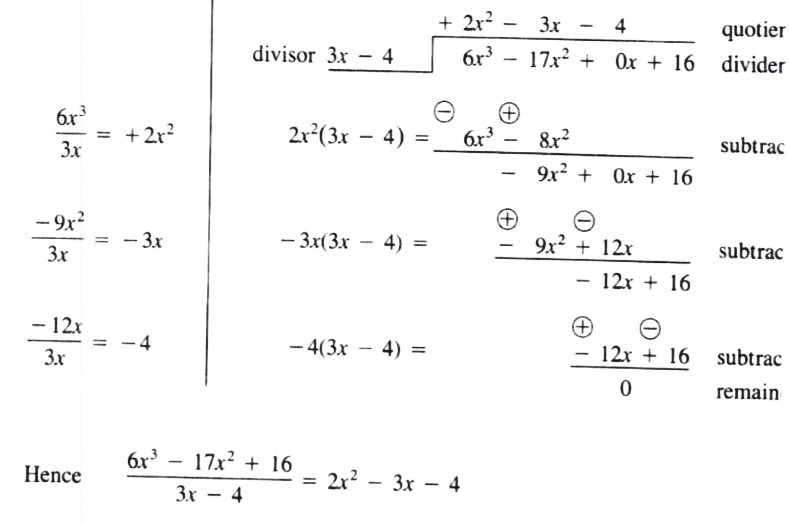EXAMPLE  Divide (19 x2-10 x3+x5-14 x+6) by (x2+1-2 x).

Solution  Write the dividend as x5+0 x4-10 x3+19 x2-14 x+6.

Write the divisor as x2-2 x+1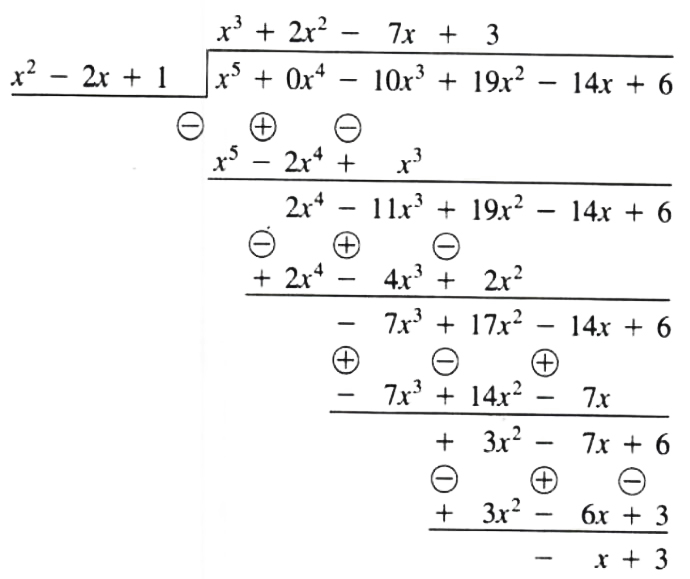Hence  x5-10 x3+19 x2-14 x+6x2-2 x+1

=x3+2 x2-7 x+3+ x+3x2-2 x+1

=x3+2 x2-7 x+3-x-3x2-2 x+1

Note  This form is similar to the form used in arithmetic when we write

207 = 2+67

EXAMPLE  Divide (2 x4-3 y4-13 x2 y2+14 xy3) by (x2+2 xy-3 y2)

Solution  Write the dividend as 2 x4+0 x3 y-13 x2 y2+14 xy3-3 y4.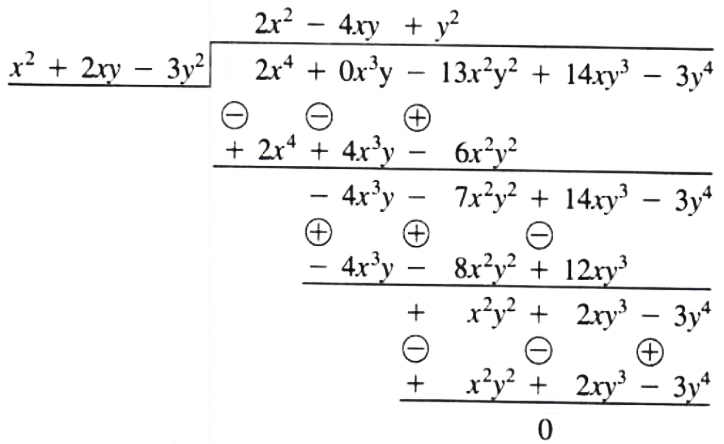Hence  2 x4-13 x2 y2+14 xy3-3 y4x2+2 xy-3 y2 = 2 x2-4 xy+y2

 ← Previous Tutorial Next Tutorial →